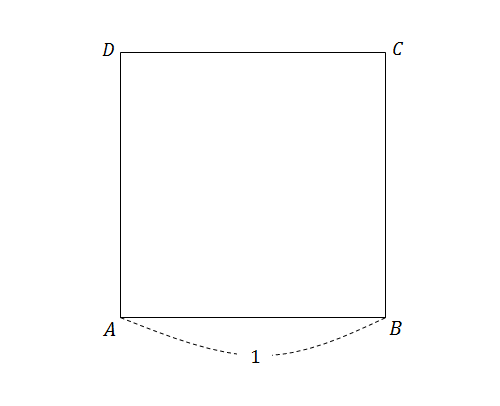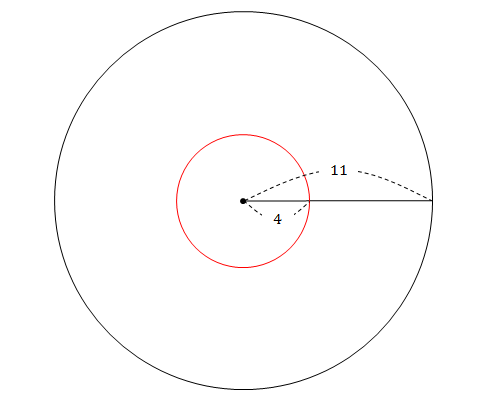Discrete Mathematics

# 2-dimensional Geometric Probability

If we randomly choose two numbers $$a$$ and $$b$$ from the interval $$[0,1],$$ what is the probability that the quadratic $$x^2+ax+b=0$$ has two distinct real roots?

What is the probability that $$x^2+ax+b=0$$ has no real roots when $$a$$ and $$b$$ are two randomly chosen numbers from the interval $$[0,2]?$$

Rachel and Ross will go to the gas station at a random time between 4 and 5 o'clock. If it takes exactly ten minutes for each person to refill his/her car and get out of the gas station, what is the probability that they meet at the gas station?In the figure above, $$\square ABCD$$ is a square with side length 1. Find the probability that $$\triangle PAB$$ is an obtuse triangle, when $$P$$ is a randomly chosen point inside $$\square ABCD.$$The figure above shows the base (of radius 11 cm) of a cylindrical bucket. There is a red circle of radius 4 cm centered at the center of the base. If Tom throws a coin of radius 1 cm into this bucket, what is the probability that the coin will land in contact with the perimeter of the red circle?

×

Problem Loading...

Note Loading...

Set Loading...